# Convolution Spatial Filtering Operations Example 3 x 3

• Slides: 20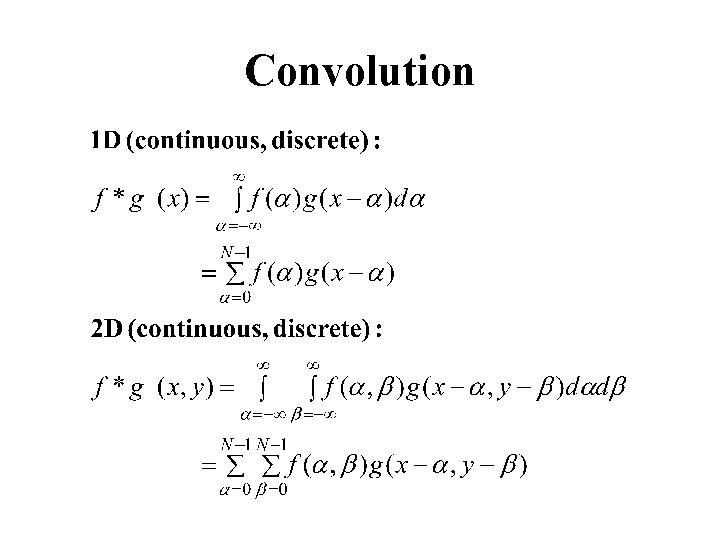ConvolutionSpatial Filtering Operations Example 3 x 3 5 x 5 g(x, y) = 1/M S f(n, m) in SNoise Cleaning Salt & Pepper Noise 3 X 3 Average 5 X 5 Average 7 X 7 Average Median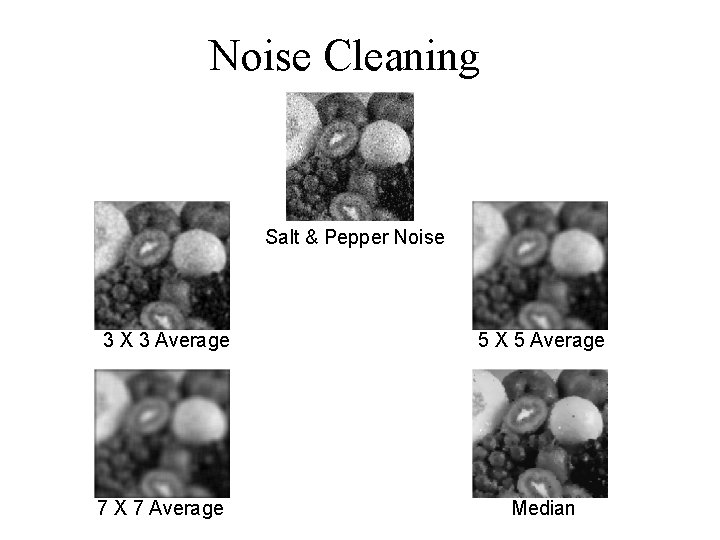Noise Cleaning Salt & Pepper Noise 3 X 3 Average 5 X 5 Average 7 X 7 Average Medianx derivative y derivative Gradient magnitude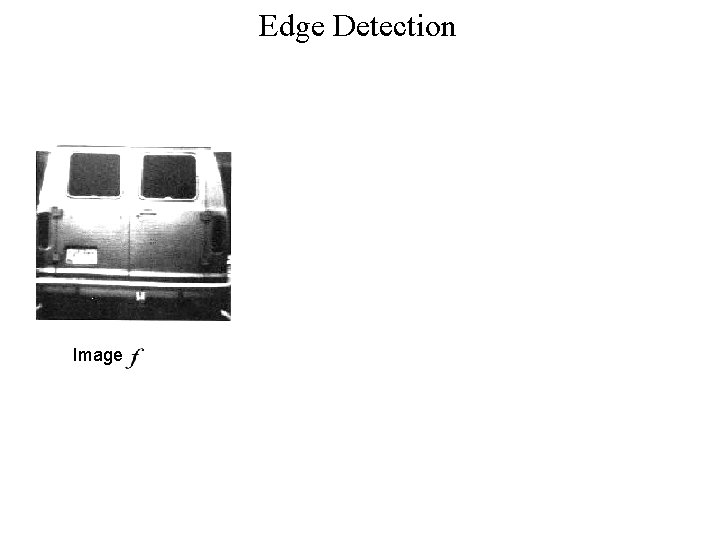Edge Detection Image Vertical edges Horizontal edgesConvolution Properties • Commutative: f*g = g*f • Associative: (f*g)*h = f*(g*h) • Homogeneous: f*( g)= f*g • Additive (Distributive): f*(g+h)= f*g+f*h • Shift-Invariant f*g(x-x 0, y-yo)= (f*g) (x-x 0, y-yo)The Convolution Theorem and similarly:Examples What is the Fourier Transform of * ?Image Domain Frequency Domain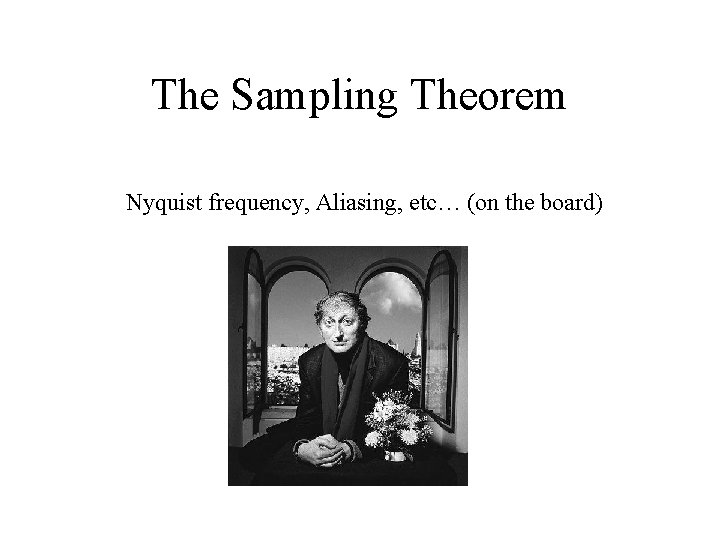The Sampling Theorem Nyquist frequency, Aliasing, etc… (on the board)Multi-Resolution Image Representation • Gaussian pyramids • Laplacian Pyramids • Wavelet Pyramids Good for: - pattern matching - motion analysis - image compression - other applications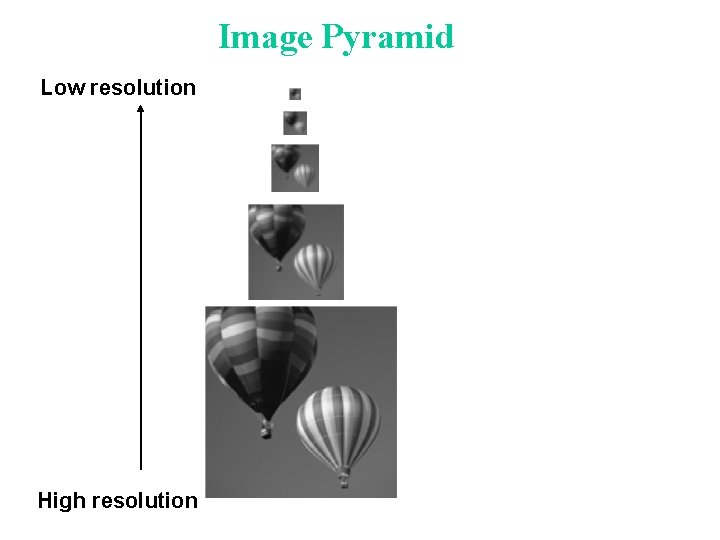Image Pyramid Low resolution High resolutionFast Pattern Matching search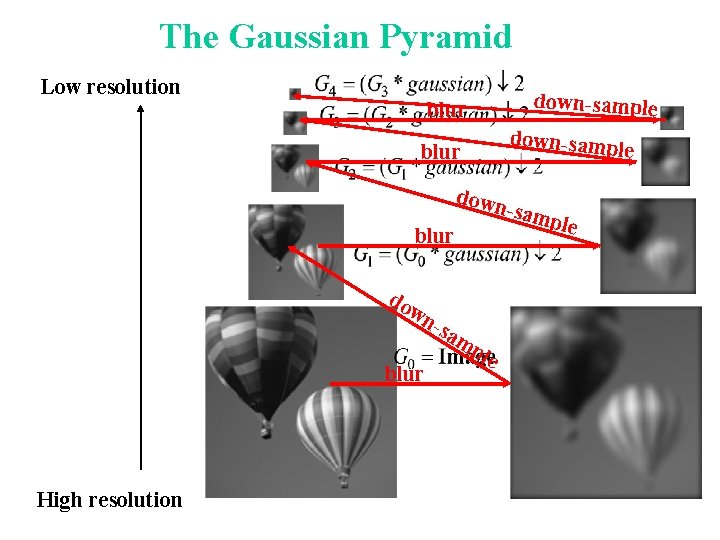The Gaussian Pyramid Low resolution down-sample blur down-samp le blur down blur do wn -sa blur High resolution mp le -sam pleThe Laplacian Pyramid Gaussian Pyramid expan - exp d and ex pa = - = nd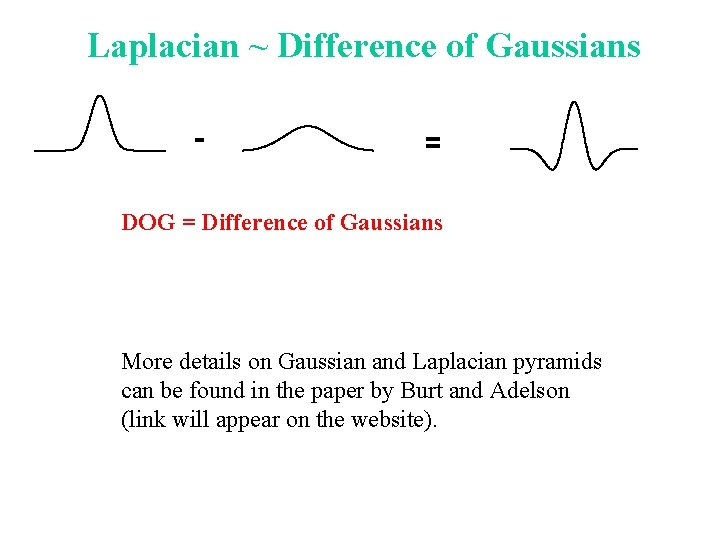Laplacian ~ Difference of Gaussians - = DOG = Difference of Gaussians More details on Gaussian and Laplacian pyramids can be found in the paper by Burt and Adelson (link will appear on the website).Computerized Tomography (CT) f(x, y) v F(u, v) uComputerized Tomography Original (simulated) 2 D image 8 projections. Frequency Domain Reconstruction from 8 projections 120 projections. Frequency Domain Reconstruction from 120 projections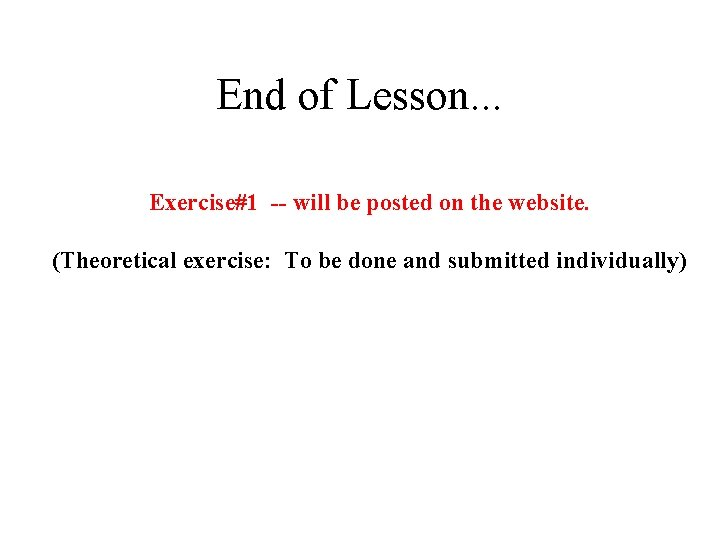End of Lesson. . . Exercise#1 -- will be posted on the website. (Theoretical exercise: To be done and submitted individually)